# Connected component labeling – Part 6

In this part of the connected component labeling series, I'll finally get to one of the algorithms actually used in the Image Processing Toolbox. It's based on a technique called union-find, as described in Sedgewick's Algorithms in C, Addison-Wesley, 1998, pp. 11-20. This algorithm is designed to be able to quickly form the union of two sets, and also to be able to quickly find which set contains a given element.

Here's the example I'll use to illustrate the method. On the left is a small binary image containing a single eight-connected component. We start by making each nonzero pixel a node in a graph. The nodes are numbered as shown on the right.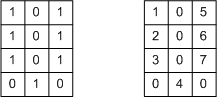In its initial state, the graph contains 7 unconnected nodes, one for each nonzero pixel: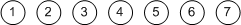We can represent this graph in MATLAB by a vector:

nodes = 1:7
nodes =

1     2     3     4     5     6     7



In this representation, nodes(k) gives the parent of node k. A node that is its own parent (that is, nodes(k) equals k) is a root node.

As we scan the image columnwise, we encounter pairs of pixels that are connected to each other. The first such pair is that pixel 2 is connected to pixel 1. We find the root node for the set containing pixel 2 (node 2) and the root node for the set containing pixel 1 (node 1). Since they are different, we form the union of those two sets simply by making the root node of one of the sets point to the root node of the other.

nodes(2) = 1
nodes =

1     1     3     4     5     6     7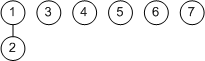The second connection encountered in the scan is that pixel 3 is connected to pixel 2. The root node for the set containing pixel 3 is node 3; the root node for the set containing pixel 2 is node 1. So we connect node 3 to node 1.

nodes(3) = 1
nodes =

1     1     1     4     5     6     7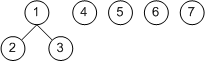Now continue to process connections. Pixel 4 is connected to pixel 3, so connect node 4 to node 1:

nodes(4) = 1
nodes =

1     1     1     1     5     6     7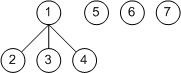Pixel 6 is connected to pixel 5, so connect node 6 to node 5:

nodes(6) = 5
nodes =

1     1     1     1     5     5     7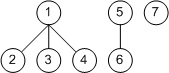Pixel 7 is connected to pixel 6, so connect node 7 to node 5:

nodes(7) = 5
nodes =

1     1     1     1     5     5     5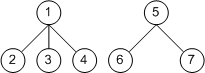Now for the moment of truth. So far our graph still contains two sets (trees). But now we see that pixel 7 is connected to pixel 4. When we connect their corresponding roots (5 to 1), we are finally left with a single set:

nodes(5) = 1
nodes =

1     1     1     1     1     5     5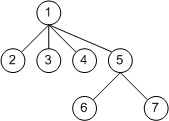According to Sedgewick, there are two important things to do to the graph while processing pairs:

• When forming a union by connecting two trees in the graph, always connect the smaller of the two trees to the larger one. This prevents the growth of long paths in the trees.
• When "walking" up a chain of nodes to find the root node, "flatten out" the tree using a technique called path compression. Here's an example showing path compression by halving. The tree on the left has a long path from node 7 up to node 1. As you follow the path, take two nodes at a time and make the bottom node point to the same parent as the top node. That give you the tree on the right.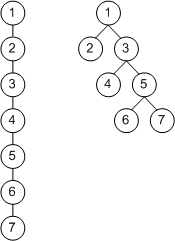If you keep doing this, then over time the trees in the graph stay almost completely flat. The flat tree is what makes the "find" operation as quick as the "union" operation.

The Image Processing Toolbox function bwlabeln, which performs connected component labeling for arbitrary dimension and arbitrary connectivity, uses the union-find technique. If you have the product, you can see the key parts of the implementation in the source files bwlabelnmex.cpp and unionfind.c, which are in toolbox\images\images\private.

I'll try to wrap this series up with one more post, which will be about the two-dimensional algorithm used by the function bwlabel.

Published with MATLAB® 7.4

|